Eight Elements Describing A Square cont…

Eight Elements Describing A Square.  On an expanding grid    (2012)Eight Elements Describing A Square 3x3Eight Elements Describing A Square 4x4Eight Elements Describing A Square 5x5Eight Elements Describing A Square 6x6Eight Elements Describing A Square 7x7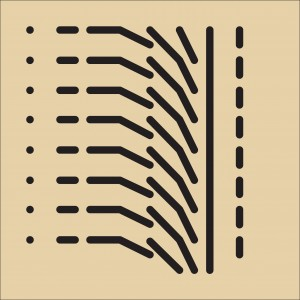Eight Elements Describing A Square 8x8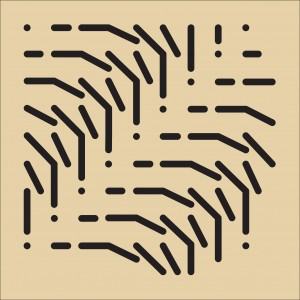Eight Elements Describing A Square 9x9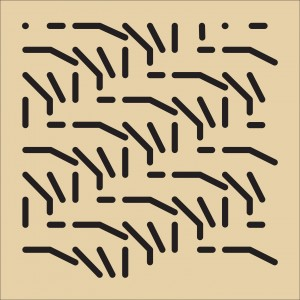Eight Elements Describing A Square 10x10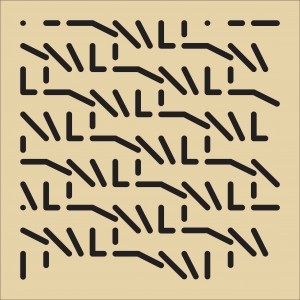Eight elements Describing A Square 11x11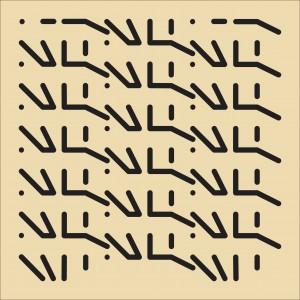Eight Elements Describing A Square 12x12